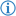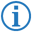The exclusive or (or either or) operator is a Boolean operator which returns `true` if and only if the number of true arguments is odd, else returns `false`.

Its token is named EXCLUSIVE_OR.

2 Inputs
Inputs Output
A B
T T F
T F T
F T T
F F F

## FDS### Example

`I did this as long as`` ``either`` ``Applejack`` ``is`` ``23`` ``or`` ``Spike`` ``is`` ``92``:` `...`

This loops the next part of code if either the variable `Applejack` is equal to `23` or the variable `Spike` is equal to `92`.

This is equivalent to the following Java code:

```while((Applejack==23)^(Spike==92)) { ...
```